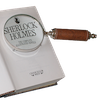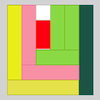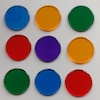# Resources tagged with: Factors and multiples

Filter by: Content type:
Age range:
Challenge level:

### There are 156 results

Broad Topics > Properties of Numbers > Factors and multiples### Mystery Matrix

##### Age 7 to 11Challenge Level

Can you fill in this table square? The numbers 2 -12 were used to generate it with just one number used twice.### Multiply Multiples 3

##### Age 7 to 11Challenge Level

Have a go at balancing this equation. Can you find different ways of doing it?### The Moons of Vuvv

##### Age 7 to 11Challenge Level

The planet of Vuvv has seven moons. Can you work out how long it is between each super-eclipse?### Multiply Multiples 1

##### Age 7 to 11Challenge Level

Can you complete this calculation by filling in the missing numbers? In how many different ways can you do it?### Multiplication Squares

##### Age 7 to 11Challenge Level

Can you work out the arrangement of the digits in the square so that the given products are correct? The numbers 1 - 9 may be used once and once only.### Multiply Multiples 2

##### Age 7 to 11Challenge Level

Can you work out some different ways to balance this equation?### Sweets in a Box

##### Age 7 to 11Challenge Level

How many different shaped boxes can you design for 36 sweets in one layer? Can you arrange the sweets so that no sweets of the same colour are next to each other in any direction?### Curious Number

##### Age 7 to 11Challenge Level

Can you order the digits from 1-3 to make a number which is divisible by 3 so when the last digit is removed it becomes a 2-figure number divisible by 2, and so on?### Tiling

##### Age 7 to 11Challenge Level

An investigation that gives you the opportunity to make and justify predictions.### It Figures

##### Age 7 to 11Challenge Level

Suppose we allow ourselves to use three numbers less than 10 and multiply them together. How many different products can you find? How do you know you've got them all?### A Mixed-up Clock

##### Age 7 to 11Challenge Level

There is a clock-face where the numbers have become all mixed up. Can you find out where all the numbers have got to from these ten statements?### Gran, How Old Are You?

##### Age 7 to 11Challenge Level

When Charlie asked his grandmother how old she is, he didn't get a straightforward reply! Can you work out how old she is?### A Square Deal

##### Age 7 to 11Challenge Level

Complete the magic square using the numbers 1 to 25 once each. Each row, column and diagonal adds up to 65.### Neighbours

##### Age 7 to 11Challenge Level

In a square in which the houses are evenly spaced, numbers 3 and 10 are opposite each other. What is the smallest and what is the largest possible number of houses in the square?### Being Resilient - Primary Number

##### Age 5 to 11Challenge Level

Number problems at primary level that may require resilience.### Number Detective

##### Age 5 to 11Challenge Level

Follow the clues to find the mystery number.### Zios and Zepts

##### Age 7 to 11Challenge Level

On the planet Vuv there are two sorts of creatures. The Zios have 3 legs and the Zepts have 7 legs. The great planetary explorer Nico counted 52 legs. How many Zios and how many Zepts were there?### Rearranged Rectangle

##### Age 7 to 11Challenge Level

How many different rectangles can you make using this set of rods?### Abundant Numbers

##### Age 7 to 11Challenge Level

48 is called an abundant number because it is less than the sum of its factors (without itself). Can you find some more abundant numbers?### Two Primes Make One Square

##### Age 7 to 11Challenge Level

Can you make square numbers by adding two prime numbers together?### Sets of Numbers

##### Age 7 to 11Challenge Level

How many different sets of numbers with at least four members can you find in the numbers in this box?### Multiples Grid

##### Age 7 to 11Challenge Level

What do the numbers shaded in blue on this hundred square have in common? What do you notice about the pink numbers? How about the shaded numbers in the other squares?### Number Tracks

##### Age 7 to 11Challenge Level

Benâ€™s class were cutting up number tracks. First they cut them into twos and added up the numbers on each piece. What patterns could they see?### Round and Round the Circle

##### Age 7 to 11Challenge Level

What happens if you join every second point on this circle? How about every third point? Try with different steps and see if you can predict what will happen.### Seven Flipped

##### Age 7 to 11Challenge Level

Investigate the smallest number of moves it takes to turn these mats upside-down if you can only turn exactly three at a time.### Fractions in a Box

##### Age 7 to 11Challenge Level

The discs for this game are kept in a flat square box with a square hole for each. Use the information to find out how many discs of each colour there are in the box.### Fitted

##### Age 7 to 11Challenge Level

Nine squares with side lengths 1, 4, 7, 8, 9, 10, 14, 15, and 18 cm can be fitted together to form a rectangle. What are the dimensions of the rectangle?### Ducking and Dividing

##### Age 7 to 11Challenge Level

Your vessel, the Starship Diophantus, has become damaged in deep space. Can you use your knowledge of times tables and some lightning reflexes to survive?### Scoring with Dice

##### Age 7 to 11Challenge Level

I throw three dice and get 5, 3 and 2. Add the scores on the three dice. What do you get? Now multiply the scores. What do you notice?### Divide it Out

##### Age 7 to 11Challenge Level

What is the lowest number which always leaves a remainder of 1 when divided by each of the numbers from 2 to 10?### Being Collaborative - Primary Number

##### Age 5 to 11Challenge Level

Number problems at primary level to work on with others.### Sets of Four Numbers

##### Age 7 to 11Challenge Level

There are ten children in Becky's group. Can you find a set of numbers for each of them? Are there any other sets?### Repeaters

##### Age 11 to 14Challenge Level

Choose any 3 digits and make a 6 digit number by repeating the 3 digits in the same order (e.g. 594594). Explain why whatever digits you choose the number will always be divisible by 7, 11 and 13.### Making Pathways

##### Age 7 to 11Challenge Level

Can you find different ways of creating paths using these paving slabs?### Dicey Array

##### Age 5 to 11Challenge Level

Watch the video of this game being played. Can you work out the rules? Which dice totals are good to get, and why?### Pebbles

##### Age 7 to 11Challenge Level

Place four pebbles on the sand in the form of a square. Keep adding as few pebbles as necessary to double the area. How many extra pebbles are added each time?### Times Tables Shifts

##### Age 7 to 11Challenge Level

In this activity, the computer chooses a times table and shifts it. Can you work out the table and the shift each time?### American Billions

##### Age 11 to 14Challenge Level

Play the divisibility game to create numbers in which the first two digits make a number divisible by 2, the first three digits make a number divisible by 3...### Playing with Factors and Multiples

##### Age 5 to 11

This article for primary teachers outlines why developing an intuitive 'feel' for numbers matters, and how our activities focusing on factors and multiples can help.### What Numbers Can We Make Now?

##### Age 11 to 14Challenge Level

Imagine we have four bags containing numbers from a sequence. What numbers can we make now?### Got it for Two

##### Age 7 to 14Challenge Level

Got It game for an adult and child. How can you play so that you know you will always win?### Big Powers

##### Age 11 to 16Challenge Level

Three people chose this as a favourite problem. It is the sort of problem that needs thinking time - but once the connection is made it gives access to many similar ideas.### Three Times Seven

##### Age 11 to 14Challenge Level

A three digit number abc is always divisible by 7 when 2a+3b+c is divisible by 7. Why?### Multiples Sudoku

##### Age 11 to 14Challenge Level

Each clue in this Sudoku is the product of the two numbers in adjacent cells.### Eminit

##### Age 11 to 14Challenge Level

The number 8888...88M9999...99 is divisible by 7 and it starts with the digit 8 repeated 50 times and ends with the digit 9 repeated 50 times. What is the value of the digit M?### Give Me Four Clues

##### Age 7 to 11Challenge Level

There are eight clues to help you find the mystery number on the grid. Four of them are helpful but the other four aren't! Can you sort out the clues and find the number?### A First Product Sudoku

##### Age 7 to 14Challenge Level

Given the products of adjacent cells, can you complete this Sudoku?### Gabriel's Problem

##### Age 11 to 14Challenge Level

Gabriel multiplied together some numbers and then erased them. Can you figure out where each number was?### Always, Sometimes or Never? Number

##### Age 7 to 11Challenge Level

Are these statements always true, sometimes true or never true?### Even So

##### Age 11 to 14Challenge Level

Find some triples of whole numbers a, b and c such that a^2 + b^2 + c^2 is a multiple of 4. Is it necessarily the case that a, b and c must all be even? If so, can you explain why?